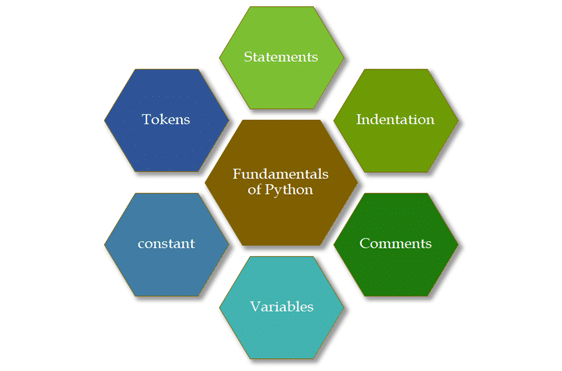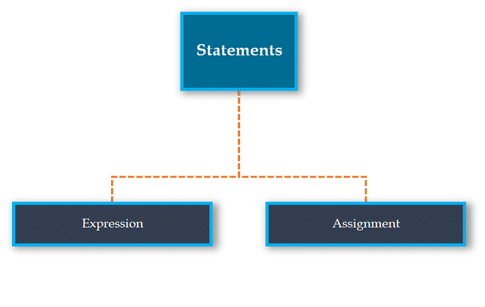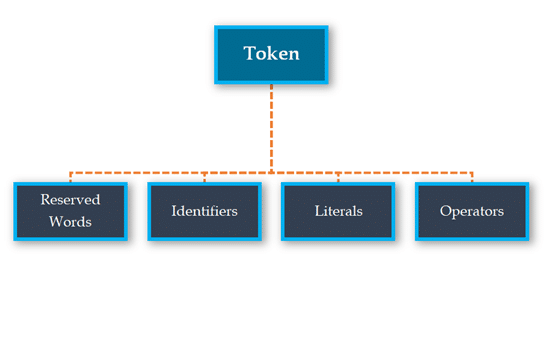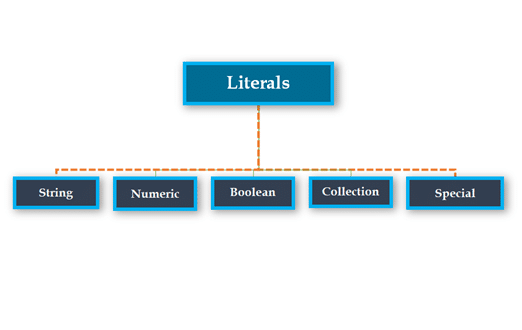# Fundamentals of Python

## Python Fundamentals:

Fundamentals of Python consists of discussion of basic building blocks of Python programming language. Here, “Fundamentals of Python” is divided into the following categories. And we will be discussing each topic separately.

• Statements
• Expressions
• Assignment Statements
• Indentations
• Multi-line comment
• Variables
• Constants
• Tokens
• Identifiers
• Keywords
• Literals
• OperatorsFirst and foremost, we will be discussing about statements in Python.

## Statements

Python statements are nothing but logical instructions that interpreter can read and execute. It can be both single and multiline.
There are two categories of statements in Python:

• Expression Statements
• Assignment Statements### Expression Statement:

With the help of expressions, we perform operations like addition, subtraction, concentration etc.
In other words, it is a statement that returns a value.
it is an expression if it appears-

• On the right side of an assignment,
• As a parameter to a method call.

Note: An expression must have a return.
Example:

• Using simple arithmetic expression:
(1+5) * 3
18
• Using a function in an expression:
pow (3,2)
9

### Assignment Statements

With the help of assignment statements, we create new variables, assign values and also change values.

### Structure of an assignment statement syntax:

#LHS <=> RHS
variable = expression

We can categorize Assignment statements in three primary categories based on what’s on the Right-Hand Side of the statement.

• Value-Based Expression on RHS
• Current Variable on RHS
• Operation on RHS

#### Value-Based Expression on the RHS:

In this case, Python allocates a new memory location to the newly assigned variable.
Let us take an example of this category.

• First, let’s create a string and assign it to a variable “test”,
• Then, we will check the memory location id python has assigned for the variable
test= “Hello World”
id(test)

Note:
Look at the example shown below:

test1=“Hello”
id(test1)
output:
2524751071304
test2=“Hello”
id(test2)
output:
2524751071304

As you might have noticed that we have assigned the same string to two different variables. But python allocated the same memory location for both the variables. That is because:
Python allocates same memory location for the two cases mentioned below:

• If the strings with less than 20 characters that don’t have white spaces and
• Integers ranging between (-5 to +255).

This concept used by Python to save memory is also called Interning.

#### Current Python variable on RHS:

In this case, Python doesn’t allocate a new memory location.
Let’s understand that with the help of an example:

current_var= “It’s HumbleGumble”
print(id(current_var))
new_var= current_var
print(id(new_var))
24751106240
2524751106240

As you can see we have the same location id allotted for the two variables.

#### Operation on RHS:

In this category, we have an operation on the right side of the statement, which is the defining factors of the type of our statement.
Let’s understand that with the help of an example:

test= 7 * 2
type(test)
int
test1= 7 * 2 / 10
type(test1)
output:
float

## Multiline statements

There are two ways to define multiline statements.

• Implicit Method
• Explicit Method

End of a statement in python is considered as a newline character, to extend the statements over multiple lines we can use two methods.

• Implicit: By using parenthesis like (), {} or [].

e.g.

a = (0 + 1 + 2 +
3 + 4 + 5)
• Explicit: By using continuation character “\”.
a = 0 + 1 + 2 + \
3 + 4 + 5

## Indentation

Unlike most of the programming languages python uses indentation to mark a block of code. According to python style guideline or PEP8, you should keep an indent size of four.Most of the programming languages provide indentation for better code formatting and don’t enforce to have it. But in Python it is mandatory. This is why indentation is so crucial in Python.

Comments are nothing but tagged lines of in codes which increases the readability of the code and make the code self-explanatory. Comments can be of two categories:

With the help of one ‘#’, we begin a single-line comment
Example:

test= 7 * 2

type(test)

#Single-line comment

With the help of ‘‘‘… ’’,’ we write multiline comments in python.
Example:

test1= 7 * 2 / 10
type(test1)
”’
line one
line two
line three
”’

Python has the documentation strings (or docstrings) feature. It gives programmers an easy way of adding quick notes with every Python module, function, class, and method.
The strings defined using triple-quotation mark are multiline comments. However, if such a string is placed immediately after a function or class definition or on top of a module, then they turn into docstrings.
Example:

def SayFunction():
”’
Strings written using ”’_”’ after a function represents docstring of func
”’
print(“just a docstring”)
print(“Let us see how to print the docstring value”)
print(theFunction.__doc__)

## Variables:

A variable is a memory address that can change and when memory address can’t change then it is known as steady. Variable is a name of the memory area where data is stored. When a variable is stored then space is distributed in memory. It characterizes a variable utilizing a combination of numbers, letters, and the underscore character.

### Assigning Values to Variables

There is no need for an explicit declaration to reserve memory. The assignment is done using the equal (=) operator. Some example of legal python variables are –

i = 1
j = 2

### Multiple Variable Assignment:

You can assign a single value to the multiple variables as follows –

a=2

Also, we can assign multiple values to the multiple variables as follows –

a, b, c = 2, 25, ‘abc’

Note: Python is type inferred language i.e. it automatically detects the type of assigned variable.
For instance,

test=1
type(test)
output:
int
test1=”String”
type(test1)
output:
str

## Constants:

Constant is a type of variable that holds values, whose value cannot be changed. In reality we rarely use constants in python.

### Assigning a value to a constant in Python

• Constants are usually declared and assigned on a different module/file.
#Declare constants in a separate file called constant.py
PI = 3.14
GRAVITY = 9.8
• Then import it to the main file.
#inside main.py we import the constants
import constant
print(constant.PI)
print(constant.GRAVITY)

## Token

Tokens are the smallest unit of the program. There are following tokens in Python:

• Reserved words or Keywords
• Identifiers
• Literals
• Operators### Keywords:

Keywords are nothing but a set of special words, which are reserved by python and have specific meanings. Remember that we are not allowed to use keywords as variables in python.
Keywords in python are case sensitive.
We’ve just captured here a snapshot of the possible Python keywords.

help> keywords
Here is a list of the Python keywords.  Enter any keyword to get more help.
False              def               if                   raise
None              del               import           return
True               elif                in                  try
and                else              is                  while
as                  except          lambda         with
assert            finally            nonlocal       yield
break             for                 not
class              from              or
continue         global           pass

### Identifiers

Identifiers in python are nothing but user-defined names to represent programmable entity like variables, functions, classes, modules or any other objects.
But there are a few rules that we need to follow while defining an identifier. They are:

• You can use a sequence of letters (lowercase (a to z) or uppercase (A to Z)). You can also mix up digits (0 to 9) or an underscore (_) while defining an identifier.
• You can’t use digits to begin an identifier name.
• You should not use Reserved Keywords to define an identifier.
• Other than underscore (_) you are not allowed to use any other special characters.
• Even though python doc says that you can name an identifier with unlimited length. But it is not entirely True.

Using a large name (more than 79 chars) would lead to the violation of a rule set by the PEP-8 standard. It says.

### Literals:

The other built-in objects in python are Literals. Literals can be defined as data that is given in a variable or constant. Python has following literals:#### String Literals:

A string literal is a sequence of characters surrounded by quotes. We can use both single, double or triple quotes for a string. And, a character literal is a single character surrounded by single or double quotes.

#### Numeric Literals:

Numeric Literals are immutable (unchangeable). Numeric literals can belong to 3 different numerical types Integer, Float, and Complex.

#### Boolean Literals:

A Boolean literal can have any of the two values: True or False.

#### Collection literals:

There are four different literal collections List literals, Tuple literals, Dict literals, and Set literals.

#### Special literals:

Python contains one special literal i.e. None. We use it to specify to that field that is not created.

### Operators:

Operators are the symbols which perform the operation on some values. These values are known as operands.
In Python, operators are categorized into the following categories:

• Arithmetic Operators
• Relational Operators
• Assignment Operators
• Logical Operators
• Membership Operators
• Identity Operators
• Bitwise Operators

#### Arithmetic Operators

 Arithmetic Operators Operator Name Description Example + Addition Perform Addition I=40, J=20 >>>I+ J >>>60 – Subtraction Perform Subtraction I=40, J=20 >>>I – J >>>20 * Multiplication Perform Multiplication I=40, J=20 >>>I * J >>> 800 / Division Perform Division I=30, J=20 >>>I /J >>> 2.5 % Modulus Return the remainder after Division I=40, J=20 >>>I /J >>> 0 ** Exponent Performs exponential (power) calculation I=4, J=20 >>>I /J >>> 204 // Floor Division Perform division remove the decimal value and return Quotient value I=30, J=20 >>>I//J >>> 1

#### Relational Operators

It is also known as comparison operator because it compares the values. After comparison, it returns the Boolean value i.e. either true or false.

 Operator Operator Name Description Example == Equal to If the values of two operands are equal, then then it returns true. I = 20, J = 20 (I == J) is True != Not Equal to If the values of two operands are not equal, then it returns true. I = 20, J = 20 (I == J) is False < Less than If the value of left operand is less than the value of right operand, then it returns true I = 40, J = 20 (I < J) is False > Greater than If the value of left operand is greater than the value of right operand, then it returns true I= 40, J = 20 (I > J) is True <= Less than or equal to If the value of left operand is less than or equal to the value of right operand, then it returns true I = 40, J = 20 (I <= J) is False >= Greater than or equal to If the value of left operand is greater than or equal to the value of right operand, then it returns true. I = 40, J = 20 (I >= J) is True <> Not equal to (similar to !=) If values of two operands are not equal, then condition becomes true I=40, J = 20 (I <> J) is True.

#### Assignment Operators

 Operator Operator Name Description Example = Assignment It assigns value from right side operand to left side operand I = 40 It assigns 40 to I += Add then assign It performs addition and then result is assigned to left hand operand I+=J that means I = I + J -= Subtract then assign It performs subtraction and then result is assigned to left hand operand I-=J that means I = I – J *= Multiply the assign It performs multiplication and then result is assigned to left hand operand. I*=J that means I = I * J /= Divide then assign It performs division and then result is assigned to left hand operand I/=J that means I = I / J %= Modulus then assign It performs modulus and then result is assigned to left hand operand I%=J that means I = I % J **= Exponent then assign It performs exponent and then result is assigned to left hand operand I**=J that means I = I ** J //= Floor division then assign It performs floor division and then result is assigned to left hand operand I//=J that means I = I // J

#### Logical Operators

 Operator Operator Name Description Example and Logical AND When Both side condition is true the result is true otherwise false 2<1 and 2<3 False or Logical OR When at least one condition is true then result is true otherwise false 2<1 or 2<3 True not Logical NOT Reverse the condition Not (5>4) False

#### Membership Operators

 Operator Description Example in It returns true if it finds a variable in the sequence otherwise returns false List = [1,2,3,4,5,6,7,8] i=1 if i in List: print(‘i is available in list’) else: print(‘i is not available in list’) Output – i is available in list not in It returns true if it does not find a variable in the sequence otherwise returns false List = [1,2,3,4,5,6,7,8] j=10 if j not in List: print (‘j is not available in list’) else: print (‘j is available in list’) Output – j is not available in list

#### Bitwise Operators

It performs bit by bit operation.
Suppose there are two variables,
I = 10 and
J = 20
and their binary values are:
I = 10 = 0000 1010
J = 20 = 0001 0100
now let us see how bitwise operators perform.

 Operator Operator Name Description Example & Binary AND If both bits are 1 then 1 otherwise 0 I & J 0000 0000 | Binary OR If one of the bit is 1 then 1 otherwise 0 I | J 0001 1110 ^ Binary XOR If both bit are same, then 0 otherwise 1 I ^ J 0001 1110 ~ Binary Complement If bit is 1 the make it 0 and if bit is 0 the make it 1 ~I 1111 0101 << Binary Left Shift The left operands is moved left by the number of bits specified by the right operand. I << 2 240 i.e. 1111 0000 >> Binary Right Shift The left operands is moved right by the number of bits specified by the right operand. I >> 2 15 i.e. 1111

#### Identity Operators

These operators are used to compare the memory address of two objects.

 Operator Description Example is It returns true if both operand ‘s identity is same otherwise false I = 20 J = 20 if(I is J): print (‘I and J have same identity’) else: print (‘I and J have not same identity’) Output – I and J have same identity is not It returns true if both operand ‘s identity is not same otherwise false I = 20 J = 230 if(I is not J): print (‘I and J have not same identity’) else: print (‘I and J have same identity’) Output – I and J have not same identity

This brings us to the end of this module.